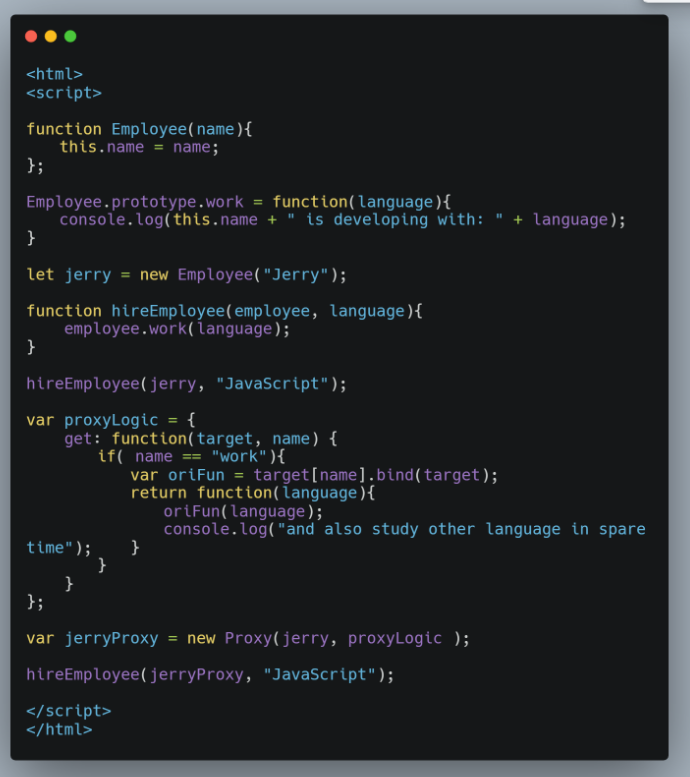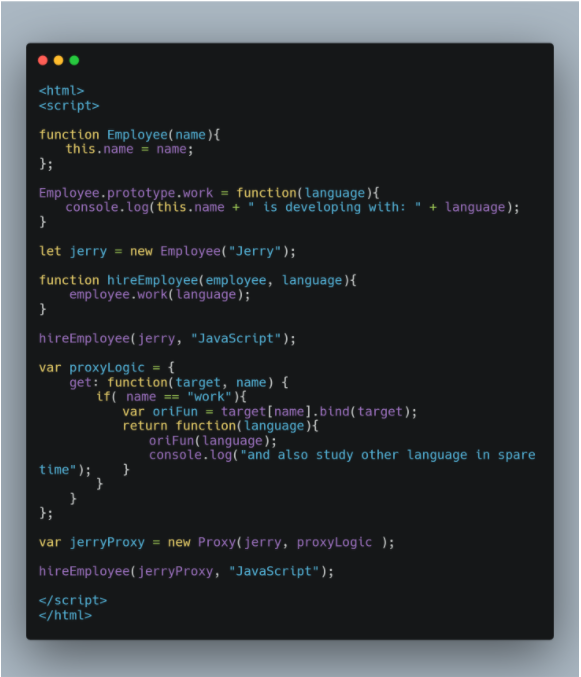# JavaScript 开发人员需要知道的简写技巧

+关注继续查看# 初级篇

## 1、三目运算符

const x = 20;
if (x > 10) {
} else {
}

const answer = x > 10 ? 'greater than 10' : 'less than 10';

## 2、循环语句

for (let i = 0; i < allImgs.length; i++)

for (let index of allImgs)

function logArrayElements(element, index, array) {
console.log("a[" + index + "] = " + element);
}
[2, 5, 9].forEach(logArrayElements);
// logs:
// a = 2
// a = 5
// a = 9

## 3、声明变量

let x;
let y;
let z = 3;

let x, y, z=3;

## 4、if 语句

if (likeJavaScript === true)

if (likeJavaScript)

## 5、十进制数

for (let i = 0; i < 10000; i++) { }

for (let i = 0; i < 1e7; i++) { }

## 6、多行字符串

const lorem = 'Lorem ipsum dolor sit amet, consectetur\n\t'
+ 'adipisicing elit, sed do eiusmod tempor incididunt\n\t'
+ 'ut labore et dolore magna aliqua. Ut enim ad minim\n\t'
+ 'veniam, quis nostrud exercitation ullamco laboris\n\t'
+ 'nisi ut aliquip ex ea commodo consequat. Duis aute\n\t'
+ 'irure dolor in reprehenderit in voluptate velit esse.\n\t'

const lorem = Lorem ipsum dolor sit amet, consectetur
adipisicing elit, sed do eiusmod tempor incididunt
ut labore et dolore magna aliqua. Ut enim ad minim
veniam, quis nostrud exercitation ullamco laboris
nisi ut aliquip ex ea commodo consequat. Duis aute
irure dolor in reprehenderit in voluptate velit esse.

# 高级篇

## 1、变量赋值

if (variable1 !== null || variable1 !== undefined || variable1 !== '') {
let variable2 = variable1;
}

const variable2 = variable1  || 'new';

let variable1;
let variable2 = variable1  || '';
console.log(variable2 === ''); // prints true
variable1 = 'foo';
variable2 = variable1  || '';
console.log(variable2); // prints foo

## 2、默认值赋值

let dbHost;
if (process.env.DB_HOST) {
dbHost = process.env.DB_HOST;
} else {
dbHost = 'localhost';
}

const dbHost = process.env.DB_HOST || 'localhost';

## 3、对象属性

ES6 提供了一个很简单的办法，来分配属性的对象。如果属性名与 key 名相同，则可以使用简写。

const obj = { x:x, y:y };

const obj = { x, y };

## 4、箭头函数

function sayHello(name) {
console.log('Hello', name);
}

setTimeout(function() {
}, 2000);

list.forEach(function(item) {
console.log(item);
});

sayHello = name => console.log('Hello', name);
list.forEach(item => console.log(item));

## 5、隐式返回值

function calcCircumference(diameter) {
return Math.PI * diameter
}

calcCircumference = diameter => (
Math.PI * diameter;
)

## 6、默认参数值

function volume(l, w, h) {
if (w === undefined)
w = 3;
if (h === undefined)
h = 4;
return l * w * h;
}

volume = (l, w = 3, h = 4 ) => (l * w * h);
volume(2) //output: 24

## 7、模板字符串

ES6 提供了相应的方法，我们可以使用反引号和 ${ } 将变量合成一个字符串。 const welcome = 'You have logged in as ' + first + ' ' + last + '.' const db = 'http://' + host + ':' + port + '/' + database; 简写为： const welcome = You have logged in as${first} ${last}; const db = http://${host}:${port}/${database};

## 8、解构赋值

const observable = require('mobx/observable');
const action = require('mobx/action');
const runInAction = require('mobx/runInAction');
const store = this.props.store;
const form = this.props.form;
const errors = this.props.errors;
const entity = this.props.entity;

import { observable, action, runInAction } from 'mobx';
const { store, form, loading, errors, entity } = this.props;

const { store, form, loading, errors, entity:contact } = this.props;

## 9、展开运算符

// joining arrays
const odd = [1, 3, 5];
const nums = [2 ,4 , 6].concat(odd);

// cloning arrays
const arr = [1, 2, 3, 4];
const arr2 = arr.slice( )

// joining arrays
const odd = [1, 3, 5 ];
const nums = [2 ,4 , 6, ...odd];
console.log(nums); // [ 2, 4, 6, 1, 3, 5 ]

// cloning arrays
const arr = [1, 2, 3, 4];
const arr2 = [...arr];

const odd = [1, 3, 5 ];
const nums = [2, ...odd, 4 , 6];

const { a, b, ...z } = { a: 1, b: 2, c: 3, d: 4 };
console.log(a) // 1
console.log(b) // 2
console.log(z) // { c: 3, d: 4 }

## 10、强制参数

function foo(bar) {
if(bar === undefined) {
throw new Error('Missing parameter!');
}
return bar;
}

mandatory = ( ) => {
throw new Error('Missing parameter!');
}
foo = (bar = mandatory( )) => {
return bar;
}

## 11、Array.find

const pets = [
{ type: 'Dog', name: 'Max'},
{ type: 'Cat', name: 'Karl'},
{ type: 'Dog', name: 'Tommy'},
]
function findDog(name) {
for(let i = 0; i<pets.length; ++i) {
if(pets[i].type === 'Dog' && pets[i].name === name) {
return pets[i];
}
}
}

pet = pets.find(pet => pet.type ==='Dog' && pet.name === 'Tommy');
console.log(pet); // { type: 'Dog', name: 'Tommy' }

## 12、Object [key]

function validate(values) {
if(!values.first)
return false;
if(!values.last)
return false;
return true;
}
console.log(validate({first:'Bruce',last:'Wayne'})); // true

// object validation rules
const schema = {
first: {
required:true
},
last: {
required:true
}
}

// universal validation function
const validate = (schema, values) => {
for(field in schema) {
if(schema[field].required) {
if(!values[field]) {
return false;
}
}
}
return true;
}
console.log(validate(schema, {first:'Bruce'})); // false
console.log(validate(schema, {first:'Bruce',last:'Wayne'})); // true

## 13、双位操作符

Math.floor(4.9) === 4  //true

~~4.9 === 4  //true

# JavaScript 开发工具推荐

SpreadJS 纯前端表格控件是基于 HTML5 的 JavaScript 电子表格和网格功能控件，提供了完备的公式引擎、排序、过滤、输入控件、数据可视化、Excel 导入/导出等功能，适用于 .NET、Java 和移动端等各平台在线编辑类 Excel 功能的表格程序开发。

# 总结67 0115 0JavaScript 开发人员应该理解的 this

55 056 0JavaScript ES6对Proxy的原生支持的一个例子：开发人员学习额外的编程语言
JavaScript ES6对Proxy的原生支持的一个例子：开发人员学习额外的编程语言
50 0JavaScript ES6对Proxy的原生支持的一个例子：开发人员学习额外的编程语言
JavaScript ES6对Proxy的原生支持的一个例子：开发人员学习额外的编程语言
50 0958 0JavaScript 开发人员更喜欢 Deno 的五大原因
NodeJS 的创造人 Ryan Dahl 刚发布了一个新的运行时 Deno，旨在解决 Node 存在的许多缺陷。像大家一样，我一开始也以为这只是又一个 JS 框架。
1168 0JavaScript 开发人员需要知道的简写技巧
1026 0IE调试网页之五：使用 F12 开发人员工具调试 JavaScript 错误 (Windows)
2226 0
cacao111

JavaScript 自学手册文档教程333265